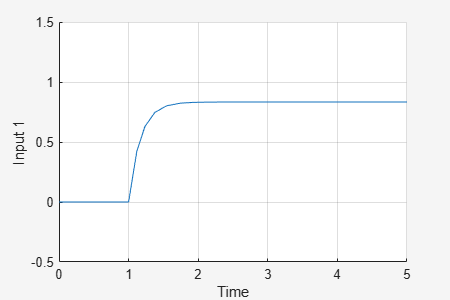# Vary Uncertain Values Across Multiple Uncertain Blocks

This example shows how to simulate a Simulink® model containing multiple Uncertain State Space blocks. You can sample all the uncertain blocks at once using the `uvars` command. This approach is useful when your model contains large numbers of uncertain variables or Uncertain State Space blocks.

### Uncertain Model

Open the model `rctMultiUncertainModel`.

```mdl = "rctMultiUncertainModel"; open_system(mdl) ```The model is contains two Uncertain State Space blocks. The Unmodeled dynamics block is preconfigured to represent uncertain dynamics with a frequency-dependent weight of the form `wt*input_unc`.

```input_unc = ultidyn('input_unc',[1 1]); wt = makeweight(0.25,130,2.5); ```

The other uncertain block is configured to represent a first-order system with an uncertain pole location.

```unc_sys = ss(ureal('a',-1,'Range',[-2 -.5]),1,5,0); ```

A step input feeds the uncertain system, and the MultiPlot Graph block shows the system.

### Simulate Nominal Model

To simulate the model, Simulink must set the uncertain parameters in both of these blocks to specific, non-uncertain values. Use the Uncertainty value parameter to specify these values. In `rctMultiUncertainModel`, both blocks are preconfigured to use the workspace variable `vals` for that parameter. To simulate the model using the nominal values of all uncertain parameters, set `vals = []`.

```vals = []; sim(mdl); ```### Generate Random Sample of All Uncertain Parameters

The `ufind` command finds all uncertain parameters in all the Uncertain State Space blocks across the entire model, and returns a structure containing their names and values.

```uvars = ufind(mdl) ```
```uvars = struct with fields: a: [1x1 ureal] input_unc: [1x1 ultidyn] ```Use `usample` to generate a random sample of the uncertain parameters in uvars. Set `vals` to this sample value.

```vals = usample(uvars) ```
```vals = struct with fields: a: -0.7779 input_unc: [1x1 ss] ```

The Uncertainty value parameter in each Uncertain State Space block is already set to `vals`. When you simulate the model, for each block, Simulink uses the value in `vals` that corresponds to the uncertain parameters in that block.

```sim(mdl) ```### Simulate Multiple Random Samples

To simulate the model at multiple random values, repeat the process of generating random values for `vals` inside a `for` loop. Each time, `usample` generates new values for the uncertain elements in the model, and the plot is updated with another step response.

```for i=1:10 vals = usample(uvars); sim(mdl); end ```